Conics:  Graphing an Ellipse

 A.  center at the origin: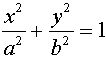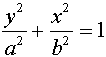B.  center at (h, k):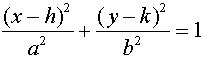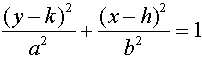Example:  Graph: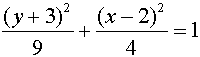Solution:  Solve the equation for y.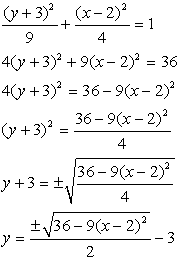Enter the equation with the positive square root into Y1. Enter the equation with the negative square root into Y2.  You cannot simply negate Y1 to obtain Y2 in this problem. OR enter using the {list} approach as shown at the right. If you choose ZOOM #6 (the standard window), remember that the viewing window has a 3/2 aspect ratio (the standard viewing screen is not a square). Choose ZOOM#5 ZSquare to create a viewing window where the units on both axes are the same length.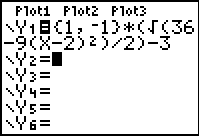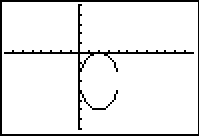NOTE:  * You may notice that the "vertical" edges of the graph may not appear in the viewing window.  The viewing screen cannot graph points that fall between pixels. * You may notice that the TRACE function will not move automatically between the two sections of the graph because the positive and negative square roots were graphed as two separate equations.  The arrow keys can be used to move between the two sections of the graph.  Also, the cursor will disappear if it is moved beyond the domain for which x is defined.